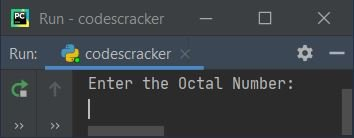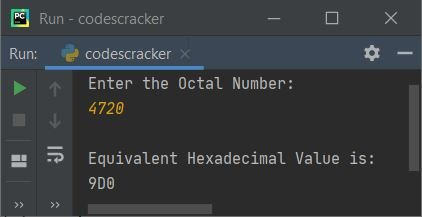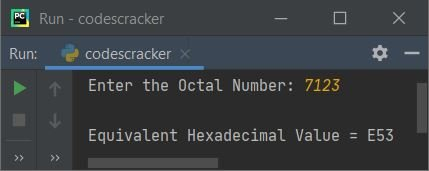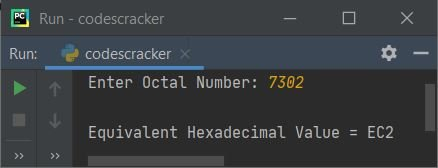# Python Program to Convert Octal to Hexadecimal

In this article, I've created some programs in Python, to convert octal number entered by user to its equivalent hexadecimal value. Here are the list of programs:

• Octal to Hexadecimal using List and while Loop
• Using String and while Loop
• Using int() and hex() Methods
• Using user-defined Function
• Using Class

Note - Before creating these programs, if you're not aware about steps used for the conversion, refer to Octal to Hexadecimal Steps and Formula to get every required things.

## Octal to Hexadecimal using List and while Loop

To convert octal to hexadecimal number in Python, you have to ask from user to enter an octal number, then convert that number into its equivalent hexadecimal value. The question is, write a Python program to convert octal number to hexadecimal using list and while loop. Here is its answer:

```print("Enter the Octal Number: ")
octnum = int(input())

chk = 0
i = 0
decnum = 0
while octnum!=0:
rem = octnum%10
if rem>7:
chk = 1
break
decnum = decnum + (rem * (8 ** i))
i = i+1
octnum = int(octnum/10)

if chk == 0:
i = 0
hexdecnum = []
while decnum != 0:
rem = decnum % 16
if rem < 10:
rem = rem + 48
else:
rem = rem + 55
rem = chr(rem)
hexdecnum.insert(i, rem)
i = i + 1
decnum = int(decnum / 16)

i = i - 1
while i >= 0:
print(end=hexdecnum[i])
i = i - 1
print()

else:
print("\nInvalid Input!")```

Here is the initial output produced by this Python program:Now supply the input say 4720 as octal number and press `ENTER` key to convert and prints its equivalent hexadecimal value as shown in the snapshot given below:In above program, the following block of code:

```while octnum!=0:
rem = octnum%10
if rem>7:
chk = 1
break
decnum = decnum + (rem * (8 ** i))
i = i+1
octnum = int(octnum/10)```

is used to convert given octal number into its equivalent decimal value. To get its in-depth working of code, refer to Octal to Decimal in Python. And the following block of code:

```while decnum != 0:
rem = decnum % 16
if rem < 10:
rem = rem + 48
else:
rem = rem + 55
rem = chr(rem)
hexdecnum.insert(i, rem)
i = i + 1
decnum = int(decnum / 16)```

is used to convert decimal to hexadecimal. To learn its in-depth working of code, refer to Decimal to Hexadecimal in Python

## Octal to Hexadecimal using String and while Loop

Now this program is created using string instead of list as used in previous program. The end= is used to skip printing of an automatic newline.

```print("Enter the Octal Number: ", end="")
onum = int(input())

chk = i = dnum = 0
while onum!=0:
rem = onum % 10
if rem>7:
chk = 1
break
dnum = dnum + (rem * (8 ** i))
i = i+1
onum = int(onum / 10)

if chk == 0:
hnum = ""
while dnum != 0:
rem = dnum % 16
if rem < 10:
rem = rem + 48
else:
rem = rem + 55
rem = chr(rem)
hnum = hnum + rem
dnum = int(dnum / 16)
hnum = hnum[::-1]

else:
print("\nInvalid Input!")```

Here is its sample run with octal number input 7123:The following statement:

`hnum = hnum[::-1]`

states that the reverse of string stored in hnum gets initialized to hnum. That is, the string stored in hnum gets reversed.

## Octal to Hexadecimal using int() and hex()

This program is created using two predefined functions namely int() and hex() to do the same job, that is of converting octal to hexadecimal. The int() returns integer equivalent of value passed as its argument. And hex() returns hexadecimal equivalent of value passed as its argument.

```print("Enter Octal Number: ", end="")
onum = input()

dnum = int(onum, 8)
hnum = hex(dnum)

Here is its sample run with user input 7302:Note - To skip first two characters, and to capitalize hex digits. Replace the following (last) statement:

`print("\nEquivalent Hexadecimal Value =", hnum)`

with new statement as given below:

`print("\nEquivalent Hexadecimal Value =", hnum[2:].upper())`

Now output with same user input as provided in previous sample run, looks like:Note - To implement user-based code for lowercase to uppercase conversion, refer to Lowercase to Uppercase in Python.

## Octal to Hexadecimal using Function

This program is created using a user-defined function named OctToHex(). This function receives a value (octal) as its argument and returns its equivalent hexadecimal value using int() and hex() methods.

```def OctToHex(o):
return hex(int(o, 8))

print("Enter Octal Number: ", end="")
onum = input()

hnum = OctToHex(onum)

## Octal to Hexadecimal using Class

This is the last program created using a class and object. That is, an object ob is created of class CodesCracker. Using this object, member function named OctToHex() gets accessed using dot (.) operator.

```class CodesCracker:
def OctToHex(self, o):
return hex(int(o, 8))

print("Enter Octal Number: ", end="")
onum = input()

ob = CodesCracker()
hnum = ob.OctToHex(onum)

#### Same Program in Other Languages

Python Online Test

« Previous Program Next Program »